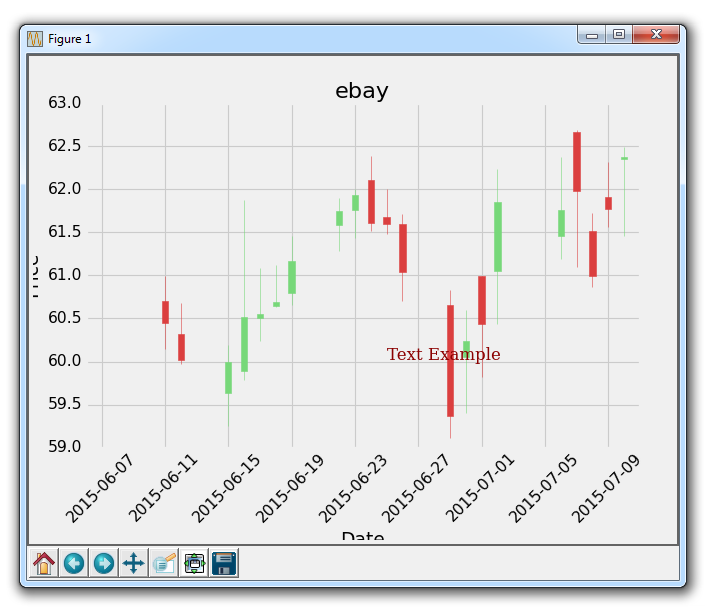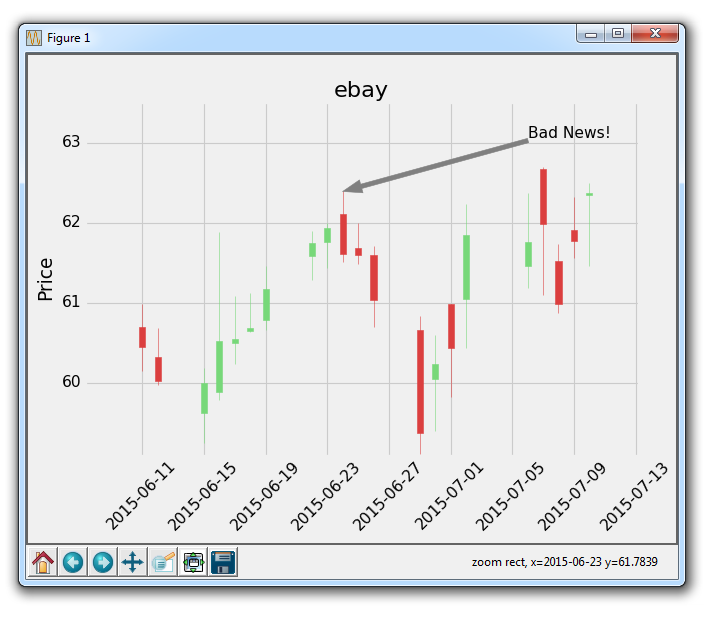# 第十七章 注解和文本

``````import matplotlib.pyplot as plt
import matplotlib.dates as mdates
import matplotlib.ticker as mticker
from matplotlib.finance import candlestick_ohlc
from matplotlib import style

import numpy as np
import urllib
import datetime as dt

style.use('fivethirtyeight')
print(plt.style.available)

print(plt.__file__)

def bytespdate2num(fmt, encoding='utf-8'):
strconverter = mdates.strpdate2num(fmt)
def bytesconverter(b):
s = b.decode(encoding)
return strconverter(s)
return bytesconverter

def graph_data(stock):

fig = plt.figure()
ax1 = plt.subplot2grid((1,1), (0,0))

stock_price_url = 'http://chartapi.finance.yahoo.com/instrument/1.0/'+stock+'/chartdata;type=quote;range=1m/csv'
stock_data = []
split_source = source_code.split('\n')
for line in split_source:
split_line = line.split(',')
if len(split_line) == 6:
if 'values' not in line and 'labels' not in line:
stock_data.append(line)

date, closep, highp, lowp, openp, volume = np.loadtxt(stock_data,
delimiter=',',
unpack=True,
converters={0: bytespdate2num('%Y%m%d')})

x = 0
y = len(date)
ohlc = []

while x < y:
append_me = date[x], openp[x], highp[x], lowp[x], closep[x], volume[x]
ohlc.append(append_me)
x+=1

candlestick_ohlc(ax1, ohlc, width=0.4, colorup='#77d879', colordown='#db3f3f')

for label in ax1.xaxis.get_ticklabels():
label.set_rotation(45)

ax1.xaxis.set_major_formatter(mdates.DateFormatter('%Y-%m-%d'))
ax1.xaxis.set_major_locator(mticker.MaxNLocator(10))
ax1.grid(True)

plt.xlabel('Date')
plt.ylabel('Price')
plt.title(stock)
plt.subplots_adjust(left=0.09, bottom=0.20, right=0.94, top=0.90, wspace=0.2, hspace=0)
plt.show()

graph_data('ebay')
``````

``````font_dict = {'family':'serif',
'color':'darkred',
'size':15}
ax1.text(date, closep,'Text Example', fontdict=font_dict)
````````````ax1.annotate('Bad News!',(date,highp),
xytext=(0.8, 0.9), textcoords='axes fraction',
arrowprops = dict(facecolor='grey',color='grey'))
````````````import matplotlib.pyplot as plt
import matplotlib.dates as mdates
import matplotlib.ticker as mticker
from matplotlib.finance import candlestick_ohlc
from matplotlib import style

import numpy as np
import urllib
import datetime as dt

style.use('fivethirtyeight')
print(plt.style.available)

print(plt.__file__)

def bytespdate2num(fmt, encoding='utf-8'):
strconverter = mdates.strpdate2num(fmt)
def bytesconverter(b):
s = b.decode(encoding)
return strconverter(s)
return bytesconverter

def graph_data(stock):

fig = plt.figure()
ax1 = plt.subplot2grid((1,1), (0,0))

stock_price_url = 'http://chartapi.finance.yahoo.com/instrument/1.0/'+stock+'/chartdata;type=quote;range=1m/csv'
stock_data = []
split_source = source_code.split('\n')
for line in split_source:
split_line = line.split(',')
if len(split_line) == 6:
if 'values' not in line and 'labels' not in line:
stock_data.append(line)

date, closep, highp, lowp, openp, volume = np.loadtxt(stock_data,
delimiter=',',
unpack=True,
converters={0: bytespdate2num('%Y%m%d')})

x = 0
y = len(date)
ohlc = []

while x < y:
append_me = date[x], openp[x], highp[x], lowp[x], closep[x], volume[x]
ohlc.append(append_me)
x+=1

candlestick_ohlc(ax1, ohlc, width=0.4, colorup='#77d879', colordown='#db3f3f')

for label in ax1.xaxis.get_ticklabels():
label.set_rotation(45)

ax1.xaxis.set_major_formatter(mdates.DateFormatter('%Y-%m-%d'))
ax1.xaxis.set_major_locator(mticker.MaxNLocator(10))
ax1.grid(True)
xytext=(0.8, 0.9), textcoords='axes fraction',
arrowprops = dict(facecolor='grey',color='grey'))

##    # Text placement example:
##    font_dict = {'family':'serif',
##                 'color':'darkred',
##                 'size':15}
##    ax1.text(date, closep,'Text Example', fontdict=font_dict)

plt.xlabel('Date')
plt.ylabel('Price')
plt.title(stock)
#plt.legend()
plt.subplots_adjust(left=0.09, bottom=0.20, right=0.94, top=0.90, wspace=0.2, hspace=0)
plt.show()

graph_data('ebay')
``````